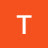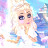Cross Multiplication | Algebra | Chegg TutorsCross Multiplication | Algebra | Chegg Tutors

Cross multiplication is a useful trick for helping you solve for a variable x in a problem involving fractions. By multiplying the numerator from one side of the equation by the denominator of the other side of the equation, you can simplify a problem by eliminating the denominators and helping you get one step closer to solving for x.

Think of the following example:

a / x = b / c, where a, b, and c are constants and x is the variable you are trying to solve for.

Using the technique of cross multiplication, you can simplify the equation by multiplying the numerators by the denominators of the other side.

a * c = b * x

The goal with cross multiplication is to help you eliminate the denominators so that you can solve for x, as this example has done. Finally, simplify the equation by moving all constants to one side of the equation and your variable, x, to the other.

x = (a * c) / b

In this video, Chegg Algebra tutor Becca R. describes how the cross multiplication technique works through a tutorial and 3 examples, ranging from low to high in difficulty.

———-

Algebra tutoring on Chegg Tutors

Learn about the Cross Multiplication technique and other algebraic techniques on Chegg Tutors. Work with live, online Algebra tutors like Becca R. who can help you at any moment, whether at 2pm or 2am.

Liked the video tutorial? Schedule lessons on-demand or schedule weekly tutoring in advance with tutors like Becca R.

———-

About Becca R., Algebra tutor on Chegg Tutors:

Michigan State University, Class of 2013
Astrophysics major

About me: I love to teach! During my years at Michigan State, I had the honor of tutoring and teaching algebra at the collegiate level for 4 years. Additionally, I was a TA for several physics labs. I’ve spent my free time advocating for public outreach; whether it’s performing chemistry demos at the Museum of Science & Industry in Tampa or giving star tours at Badlands National Park. You can expect a thorough, enthusiastic lesson!

Want to book a private lesson with Becca R.?

———-

Like what you see? Subscribe to Chegg’s Youtube Channel:

http://bit.ly/1PwMn3k

———-

Visit Chegg.com for purchasing or renting textbooks, getting homework help, finding an online tutor, applying for scholarships and internships, discovering colleges, and more!

https://chegg.com

———-

Want more from Chegg? Follow Chegg on social media:

http://instagram.com/chegg

algebra tutors,Chegg,Chegg Study,Online Tutoring,Homework Help,Student Hub,Education,EdTech,Cross Multiplication,Algebra,Multiplication,Variables,Solve,Solve for x,Tutorial,Tutors,Tutoring

algebratutors.org

1.Theresa Sutherland says:
2.GameWithCoco says: